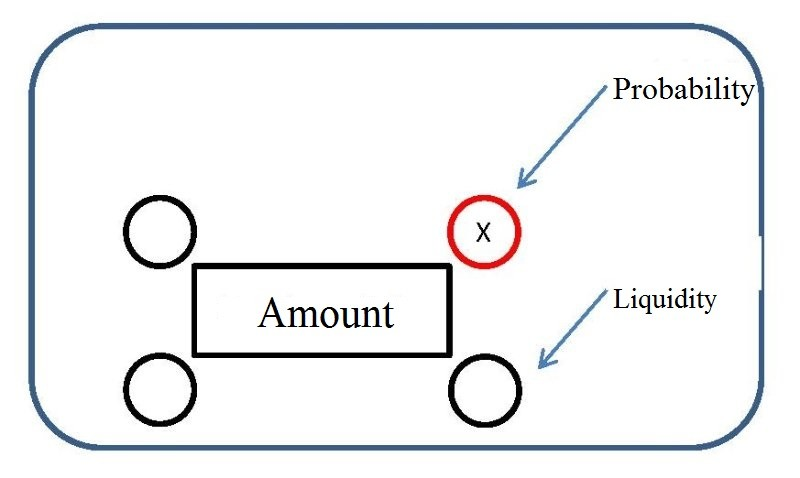## Montant relatif – Partie 1 : Indice de probabilité### Principle

The purpose of financial projections is to estimate the future performance of a particular issuer using the necessary assumptions in order to prepare the budget decisions, to draw up a financing plan or to guide the buyer in his subscription.

These financial projections are calculated using more or less known future data and some at the time of projection.

Including only future data projections without further details, the financial projection does not provide all the expected informations and therefore does not allow the buyer to have an overall view of the future performance of an issuer. The buyer does not know the probability that the data specified in the projection is real at the time “T + 1”.The relative amount allows this through its various indices.

The probability index allows to show an index (X) indicating according to certain criteria (cf.§2) the probability of realizing the amount entered in the cell at time T + 1. Thus, investors or all interested people in the financial projection will be able (in addition to the currency amount) to know the percentage of the probability that this amount will truly be held by the issuer at the time “T + 1”.

### Criteria of the Probability Index

The probability index of a cell is calculated according to the amount stipulated in the projection is more or less guaranteed at the day of the calculation of this one.

The amount entered in the cell is guaranteed by:

• Issuer’s opinion: 10% index (X = 0.1)
• Opinion of an independent party: 20% index (X = 0.2)
• Letter of intent: 30% index (X = 0.3)
• Letter of intent + evidence of funds: 40% index (X = 0.4)
• Fixed contract: 50% index (X = 0.5)
• Fixed contract + evidence of funds: 60% index (X = 0.6)
• Clear order of funds: 70% index (X = 0.7)
• Bank guarantee: 80% index (X = 0.8)
• Debt securities: 90% index (X = 0.9)
• Cash: 100% index (X = 1)

### Use of the probability index:

The index of probability adds up according to the different guarantees which composed it.

Ex: The “profit” cell is \$ 400. In these \$ 400, half comes from the opinion of an independent party (20% probability) and the other half is in cash already in possession of the issuer (100% probability). The index X will therefore be 0.60.

The probability index is weighted according to the different guarantees which composed it.

Ex: The “profit” cell is \$ 400. In these \$ 400, \$ 100 comes from the opinions of an independent party (20% probability) and the remaining \$ 300 comes from a bank guarantee (80% probability). The index X will therefore be 0.65.